# AC Power

As in the case with DC power, the instantaneous electric power in an AC circuit is given by P = VI, but these quantities are continuously varying. Almost always the desired power in an AC circuit is the average power, which is given by

Pavg = VI cosφ

where φ is the phase angle between the current and the voltage and where V and I are understood to be the effective or rms values of the voltage and current. The term cos φ is called the "power factor" for the circuit.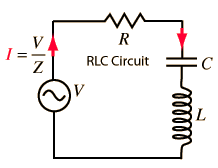RLC series circuit details
 For C = μF
 and L = mH
 and resistance R = ohms
 at angular frequency ω = x10^ rad/s,
 frequency f = x10^ Hz = kHz = MHz

the impedance is

 Z = x10^ ohms at phase φ = degrees.

For an applied rms voltage V = volts,

the rms current will be I = x 10^ amperes.

 and the AC power is given by Pavg = VI cosφ = watts

 The power factor is cos φ =
so the power is reduced to that fraction of what it would be in a DC circuit with the same voltage and current.

Default values will be entered for unspecified parameters, but all component values can be changed. Click outside the box after entering data to initiate the calculation.
Index

AC Circuits

 HyperPhysics*****Electricity and magnetism R Nave
Go Back

# Instantaneous Power

As in DC circuits, the instantaneous electric power in an AC circuit is given by P=VI where V and I are the instantaneous voltage and current.
 Sincethen the instantaneous power at any time t can be expressed as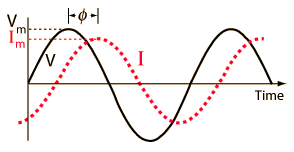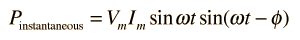and using the trig identitythe power becomes: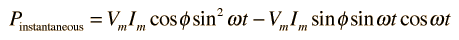Averaging this power over a complete cycle gives the average power.

Index

AC Circuits

 HyperPhysics*****Electricity and magnetism R Nave
Go Back

# Average Power

Normally the average power is the power of interest in AC circuits. Since the expression for the instantaneous poweris a continuously varying one with time, the average must be obtained by integration. Averaging over one period T of the sinusoidal function will give the average power. The second term in the power expression above averages to zero since it is an odd function of t. The average of the first term is given byShow
 Since the rms voltage and current are given byand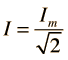,
the average power can be expressed as
Pavg = VI cosφ
Index

AC Circuits

 HyperPhysics*****Electricity and magnetism R Nave
Go Back

# Average Power Integral

Finding the value of the average power for sinusoidal voltages involves the integralThe period T of the sinusoid is related to the angular frequency ω and angle θ by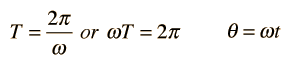Using these relationships, the integral above can be recast in the form: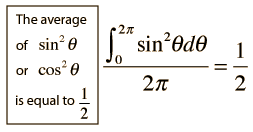Which can be shown using the trig identity: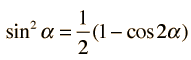which reduces the integral to the value 1/2 since the second term on the right has an integral of zero over the full period.
 More detail on integrating trig functions
Index

AC Circuits

 HyperPhysics*****Electricity and magnetism R Nave
Go Back